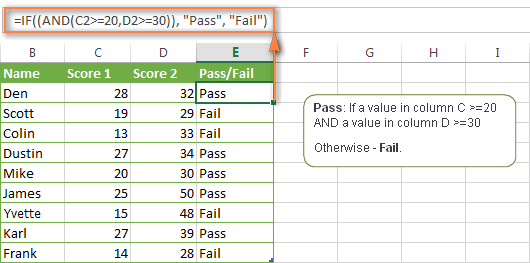I am trying to use a simple IF formula in a cell but I get This is not a formula error.

This is the IF I am trying (C6 is empty):

```=IF(C6>=70,"Pass","Fail")
```

What I really need is a formula that calculate multiplication between two cells or empty cells depending on value of one of the two cells.
Something like:

```=IF(G12=1,D2*G12,"")
```

Apr 5, 2022 in Database 1,175 views

## 1 answer to this question.

Using Excel's IF & AND function Assume you have a table containing the results of two exams. The initial score, which is stored in column C, must be at least 20. The second score, which appears in column D, must be at least 30. A student passes the final test only if both of the above conditions are met.

The simplest approach to create a suitable formula is to write out the condition first, then incorporate it into your IF function's logical test argument:

AND(B2>=20, C2>=30) is a condition.

The IF/AND formula is as follows:

=IF((AND(C2>=20, D2>=30)), "Pass", "Fail")• 23,220 points

## If Cell Starts with Text String... Formula

I have formula that checks if the ...READ MORE

## MS Excel - SumProduct formula with Loop

Drag this to the right of cell ...READ MORE

## Prevent cell numbers from incrementing in a formula in Excel

In Excel, you can use a feature ...READ MORE

## Excel formula to remove comma, spaces, period and add a text

The steps to accomplish this are as ...READ MORE

## Remove special characters from the specified string in excel

To erase a specific character from a ...READ MORE

## Remove duplicates within a row

To check for duplicates, choose the cells ...READ MORE

## EXCEL: How to automatically add serial number in Excel Table using formula that is immune to filtering / sorting?

That's a great question, and it's a ...READ MORE